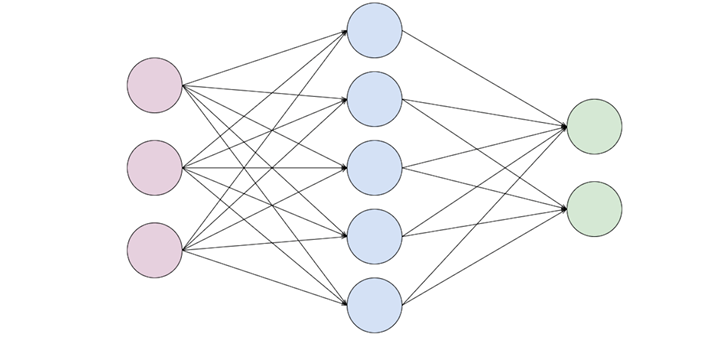Python Tutorial

# Sort dictionary by key/value in Python

To sort a dictionary by its values in Python, you can use the sorted() function along with a lambda function as the key argument. The lambda function is used to extract the values from the dictionary, and sorted() returns a new list of tuples containing the key-value pairs sorted based on the values.

# define a dictionary color_dict = {'red': 10, 'blue': 5, 'green': 20, 'yello': 15} # sort the dictionary by value in ascending order sorted_dict_asc = dict(sorted(color_dict.items(), key=lambda x: x)) # sort the dictionary by value in descending order sorted_dict_desc = dict(sorted(color_dict.items(), key=lambda x: x, reverse=True)) # print the sorted dictionaries print(sorted_dict_asc) print(sorted_dict_desc)
# Output: {'blue': 5, 'red': 10, 'yello': 15, 'green': 20} {'green': 20, 'yello': 15, 'red': 10, 'blue': 5}

In the above example, two sorted dictionaries are created: sorted_dict_asc is sorted by value in ascending order, and sorted_dict_desc is sorted by value in descending order. The sorted function is used with a lambda function as the key argument to specify the sorting criterion. The lambda function takes each key-value pair as an input (x) and returns its value (x). If the reverse parameter is True, the sorting is done in descending order, otherwise it's done in ascending order. Finally, the sorted key-value pairs are converted back into dictionaries using the dict function.

## Sort a dictionary by key | Ascending/Descending

To sort a dictionary by its keys in either ascending or descending order, you can effectively utilize the sorted() function, employing the items() method of the dictionary as the argument. By specifying the reverse parameter as True for descending order or False for ascending order, you can achieve the desired sorting outcome. This approach allows for efficient and flexible sorting of dictionary contents based on their keys, providing developers with a straightforward and powerful tool for data manipulation and presentation. Here's an example:

# define a dictionary color_dict = {'red': 10, 'blue': 5, 'green': 20, 'yello': 15} # sort the dictionary by key in ascending order sorted_dict_asc = dict(sorted(color_dict.items())) # sort the dictionary by key in descending order sorted_dict_desc = dict(sorted(color_dict.items(), reverse=True)) # print the sorted dictionaries print(sorted_dict_asc) print(sorted_dict_desc)
# Output: {'blue': 5, 'green': 20, 'red': 10, 'yello': 15} {'yello': 15, 'red': 10, 'green': 20, 'blue': 5}

In the above example, two sorted dictionaries are created: sorted_dict_asc is sorted by key in ascending order, and sorted_dict_desc is sorted by key in descending order. The sorted function is used with the items method of the dictionary as the argument to specify the sorting criterion. If the reverse parameter is True, the sorting is done in descending order, otherwise it's done in ascending order. Finally, the sorted key-value pairs are converted back into dictionaries using the dict function.

## How dictionary Sorting Algorithm works

The sorting algorithm for dictionaries in Python works differently for sorting by keys versus sorting by values.When sorting a dictionary by keys, Python follows a straightforward process of iterating over the dictionary keys and sorting them based on the specified criteria. This can be achieved using the sorted() function, which returns a list of the sorted keys. On the other hand, when sorting a dictionary by values, the process is more involved due to the inherent unordered nature of dictionaries. The values need to be extracted and sorted independently, typically using the items() method to create a list of key-value pairs.

Once sorted, these pairs can be used to create a new dictionary with the desired order. The comparison function used by the sorted() function can be customized using the key argument to achieve more sophisticated sorting behavior based on keys or values. Your explanation provides valuable insights into the sorting techniques used in Python dictionaries, making it a helpful guide for developers seeking to understand and manipulate dictionary data efficiently.

### Conclusion

You can sort a dictionary by its keys or values using the sorted() function. To sort by keys, simply pass the dictionary keys as the argument to sorted(), and for sorting by values, use the items() method to create a list of key-value pairs and then sort based on the values. The sorted() function provides a flexible and efficient way to organize the elements of a dictionary in either ascending or descending order based on the specified criteria.